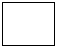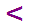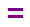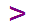Skill 6A
< > = Comparing Numbers to 30

Your student must understand the true meaning of the less than, more than and equals symbols.  They should not be using the crutch commonly taught in 1st grade which is - "the bigger end of the symbol faces the bigger number."  In other words, your student must be able to read through the number sentence from left to right and verbally say the the number and then either " is less than," "is more than," or "is equal to,"  and then the number on the right.   If it takes longer than 5 seconds to do this, then the skill absolutely must be practiced!

Below each problem are three symbols used to compare numbers.  I want you to read the number sentence and use either the words -
is more than, is less than, or is equal to to make the number sentence TRUE.  Then point to the correct symbol below the number sentence.
Again, if your student cannot do exactly this, the skill must be practiced. It is not sufficient if your student just points to the correct symbol.

 12825251330